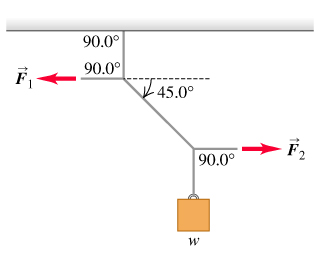# Problem: In the figure the weight w is 60.1 N. (a) What is the tension in the diagonal string? (b) Find the magnitudes of the horizontal forces F1 and F2 that must be applied to hold the system in the position shown.

###### FREE Expert Solution
89% (363 ratings)
###### Problem Details

In the figure the weight w is 60.1 N. (a) What is the tension in the diagonal string? (b) Find the magnitudes of the horizontal forces F1 and F2 that must be applied to hold the system in the position shown.Frequently Asked Questions

What scientific concept do you need to know in order to solve this problem?

Our tutors have indicated that to solve this problem you will need to apply the Equilibrium in 2D concept. You can view video lessons to learn Equilibrium in 2D. Or if you need more Equilibrium in 2D practice, you can also practice Equilibrium in 2D practice problems.

How long does this problem take to solve?

Our expert Physics tutor, Julia took 3 minutes and 57 seconds to solve this problem. You can follow their steps in the video explanation above.

What professor is this problem relevant for?

Based on our data, we think this problem is relevant for Professor Dutta's class at VANDERBILT.